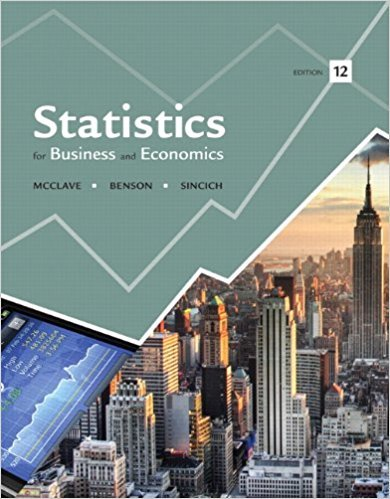×
Get Full Access to Statistics For Business And Economics - 12 Edition - Chapter 6 - Problem 4e
Get Full Access to Statistics For Business And Economics - 12 Edition - Chapter 6 - Problem 4e

×

# A random sample of 90 observations produced a mean x?=ISBN: 9780321826237 51

## Solution for problem 4E Chapter 6

Statistics for Business and Economics | 12th Edition

• Textbook Solutions
• 2901 Step-by-step solutions solved by professors and subject experts
• Get 24/7 help from StudySoup virtual teaching assistantsStatistics for Business and Economics | 12th Edition

4 5 1 392 Reviews
29
0
Problem 4E

Problem 4E

A random sample of 90 observations produced a mean x̄= 25.9 and a standard deviation s= 2.7.

a. Find an approximate 95% confidence interval for the population mean μ.

b. Find an approximate 90% confidence interval for μ.

c. Find an approximate 99% confidence interval for μ.

Step-by-Step Solution:

Step 1 of 3:

Given,a). To find an approximate 95% confidence interval for the population meanFor confidence coefficient 0.95,and(using the standard normal table, the z value for 95% CI is 1.96)

That is,The confidence interval is(25.34, 26.46)

Therefore, the confidence interval is (25.34, 26.46).

Step 2 of 3

Step 3 of 3

##### ISBN: 9780321826237

Statistics for Business and Economics was written by and is associated to the ISBN: 9780321826237. The full step-by-step solution to problem: 4E from chapter: 6 was answered by , our top Business solution expert on 07/21/17, 05:42AM. Since the solution to 4E from 6 chapter was answered, more than 700 students have viewed the full step-by-step answer. This full solution covers the following key subjects: approximate, confidence, interval, ind, mean. This expansive textbook survival guide covers 15 chapters, and 1631 solutions. The answer to “A random sample of 90 observations produced a mean x?= 25.9 and a standard deviation s= 2.7.a. Find an approximate 95% confidence interval for the population mean ?.b. Find an approximate 90% confidence interval for ?.c. Find an approximate 99% confidence interval for ?.” is broken down into a number of easy to follow steps, and 44 words. This textbook survival guide was created for the textbook: Statistics for Business and Economics , edition: 12.

Unlock Textbook Solution